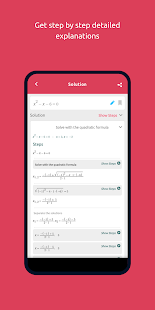Your private math tutor, solves any math problem with steps!

Your private math tutor, solves any math problem with steps! equations, integrals, derivatives, , limits and much more.
(Steps require a one-time in-app purchase)

Symbolab Math Solver app is composed of over a hundred Symbolab most powerful calculators:
Equation Calculator
Integral Calculator
Derivative Calculator
Limit calculator
Inequality Calculator
Trigonometry Calculator
Matrix Calculator
Functions Calculator
Series Calculator
ODE Calculator
Laplace Transform Calculator

Symbolab Math Solver solves any math problem including Pre- Algebra, Algebra, Pre-Calculus, Calculus, Trigonometry, Functions, Matrix, Vectors, Geometry and Statistics.

What’s New:
– Personalize your account with avatars
– Enhancements and bugfixes

★ PRO / Paid features Unlocked
★ Unwanted Permissions + Activities removed
★ Unwanted Services + Receivers and Providers removed
★ Ads Activities + Services and Providers removed
★ Debug code removed
★ Original package signature changed
➡ Languages: Multi Languages
➡ CPU architectures: arm64-v8a, armeabi-v7a, x86, x86_64
➡ Screen DPIs: 120dpi, 160dpi, 240dpi, 320dpi, 480dpi, 640dpi

Screenshots

••••